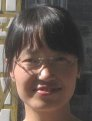Electron. J. Diff. Equ., Vol. 2011 (2011), No. 66, pp. 1-19.

### Positive periodic solutions for third-order nonlinear differential equations Jingli Ren, Stefan Siegmund, Yueli Chen

Abstract:
For several classes of third-order constant coefficient linear differential equations we obtain existence and uniqueness of periodic solutions utilizing explicit Green's functions. We discuss an iteration method for constant coefficient nonlinear differential equations and provide new conditions for the existence of periodic positive solutions for third-order time-varying nonlinear and neutral differential equations.

Submitted April 19, 2011. Published May 18, 2011.
Math Subject Classifications: 34B18, 34B27, 35B10
Key Words: Green's function; positive periodic solution; third-order differential equation.

Show me the PDF file (58 KB), TEX file, and other files for this article.Jingli Ren Department of Mathematics, Zhengzhou University Zhengzhou 450001, China. Department of Mathematics, Dresden University of Technology Dresden 01062, Germany email: renjl@zzu.edu.cn Stefan Siegmund Department of Mathematics, Dresden University of Technology Dresden 01062, Germany email: stefan.siegmund@tu-dresden.de Yueli Chen Department of Mathematics, Zhengzhou University Zhengzhou 450001, China